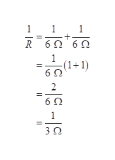# the following formula is used in physics for parallel resistance 1/R=1/r1+1/r2 find the parallel resistance, R when R1 and R2 are each 6 ohms

Question
58 views

the following formula is used in physics for parallel resistance
1/R=1/r1+1/r2
find the parallel resistance, R when R1 and R2 are each 6 ohms

check_circle

Step 1

The formula for the equivalent resistance of the resistors connected in parallel combination is:

Step 2

Substitute the known va...help_outlineImage Transcriptionclose+ R6Ω' 6 0 60 1 1+ 1 6Ω 2 6Ω 1 3 0 fullscreen

### Want to see the full answer?

See Solution

#### Want to see this answer and more?

Solutions are written by subject experts who are available 24/7. Questions are typically answered within 1 hour.*

See Solution
*Response times may vary by subject and question.
Tagged in